# Slide it down!

The last example we prepared for you will be about the sliders on your Synthia.

The purpose of this code is to have the left slider turn on and off the LEDs in the Track matrix and the right slider turn on and off the LEDs in the Sliders matrix.

To do so, we must first set the values of the left and right sliders.

Let's create two variables and call them "val_left" and "val_right"The value will be determined by the position of your sliders at the time.

Now, let's see what happens when sliders move.

You'll need I/O blocks for that.When we move the slider, its value changes to reflect its current position.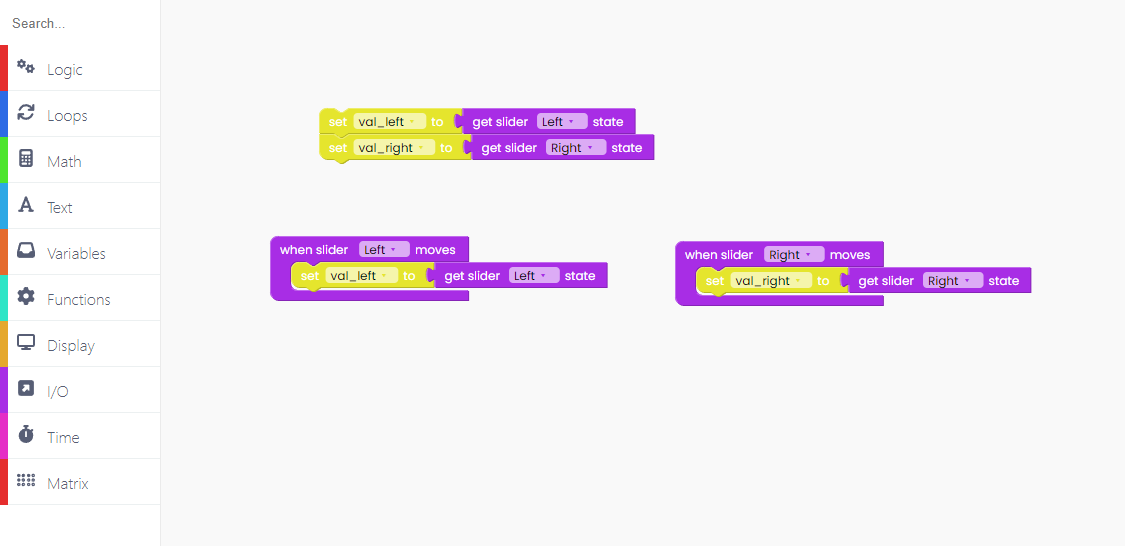Let's code the part that will be happening all the time, in loops.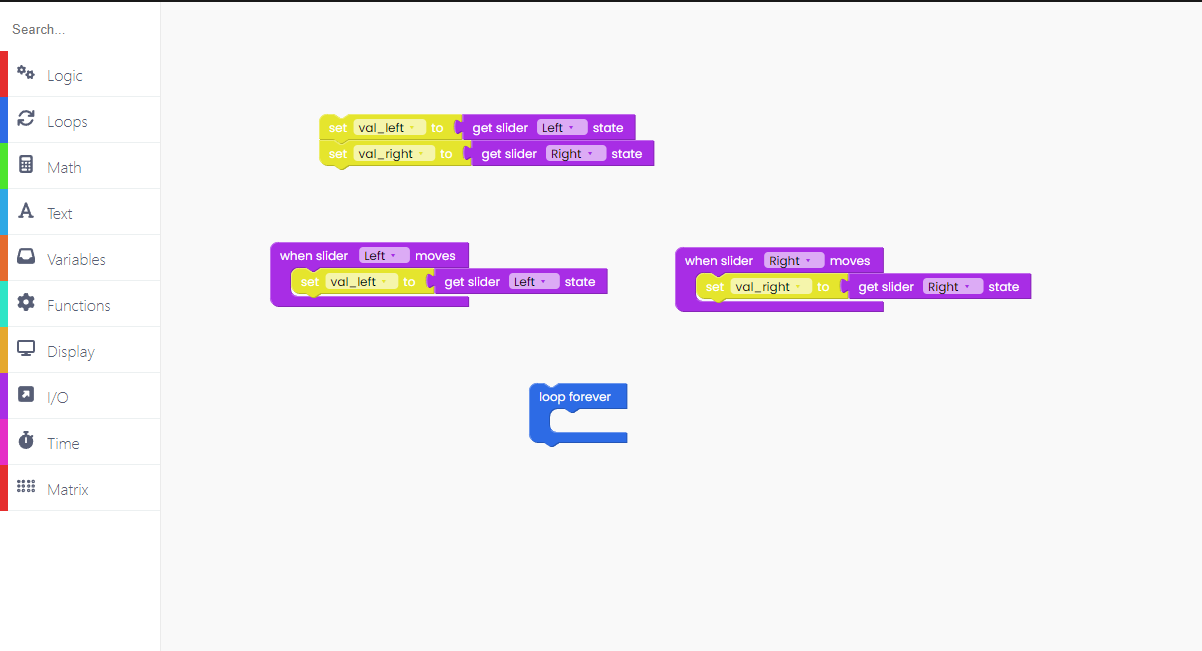As always, the initial step is to clear one matrix.

For that, we'll need the "fill matrix frame with 0".

The first matrix we'll work on is the Track matrix.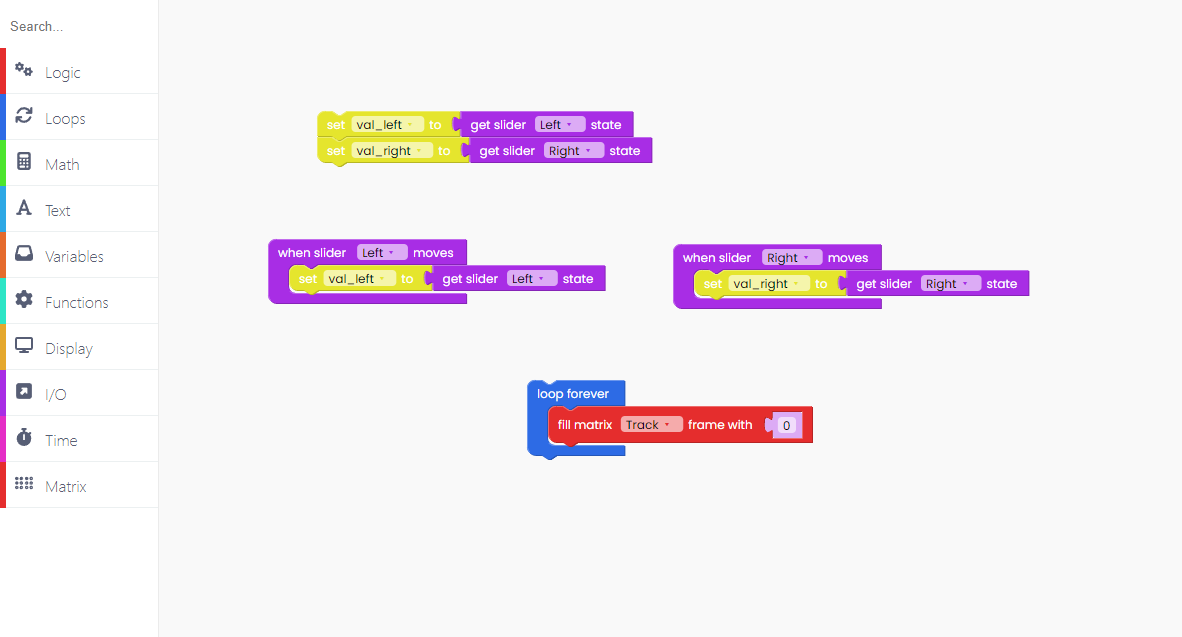Now, let's draw a filled rectangle in the Track matrix.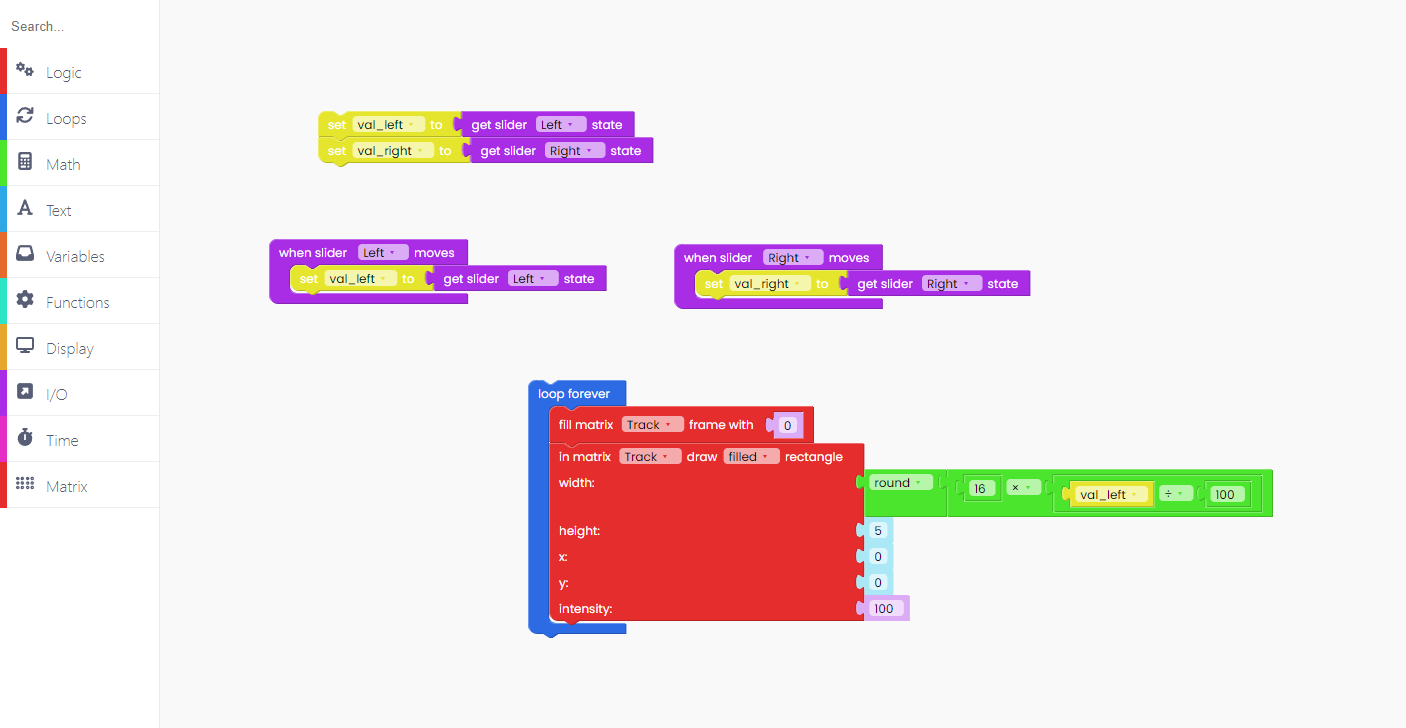The LED intensity is set to 100, and the height is set to 5 (maximum).

The width is set to the relative number equal to this math operation.

The maximum width of the matrix is 16, which we must divide by 100 because the sliders have a range of 0 to 100.

To ensure that this rectangle appears, add the "push frame to matrix" block.Now, let's do the same for the Sliders matrix.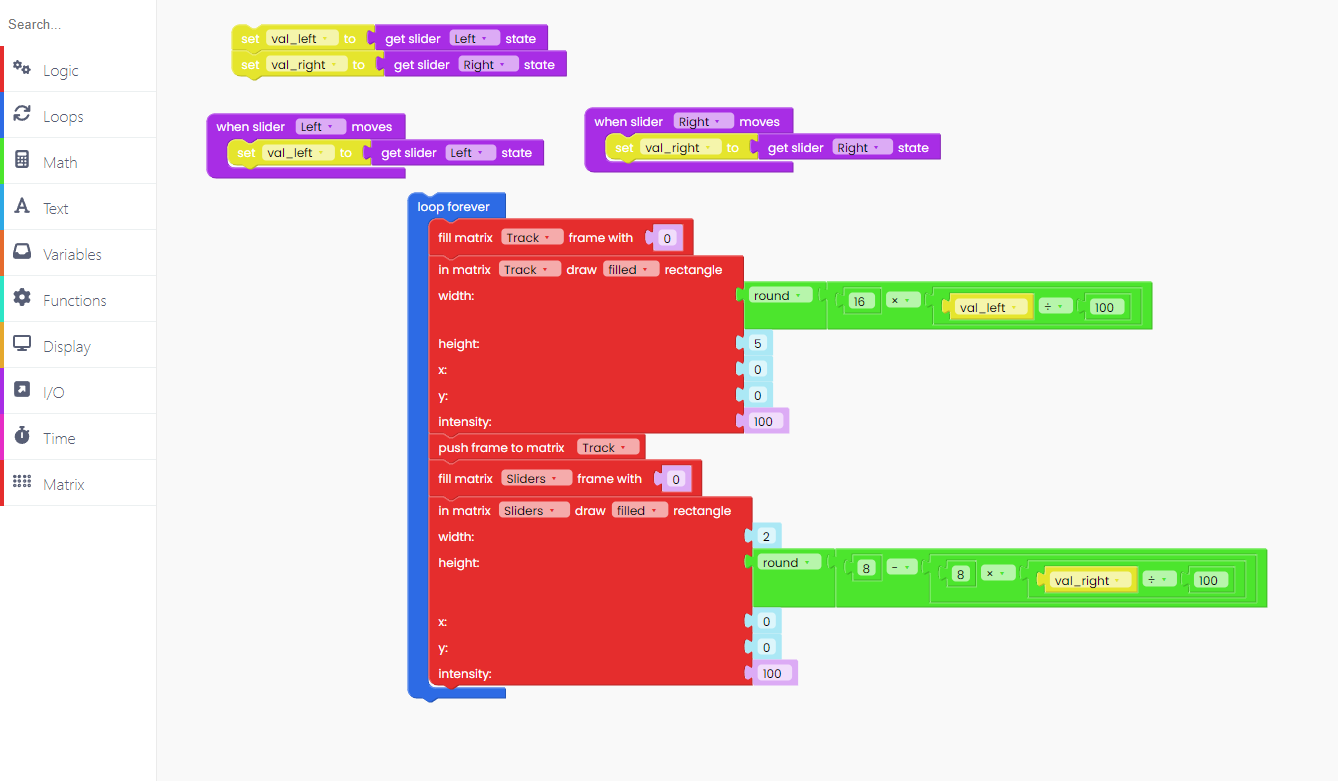We adjusted the height and width this time.

Remember to include the "push frame to matrix" and "scan sliders" blocks at the end.

Click on the Run button and check it out.